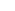SOME NEW LAWS FOR THE SOLAR SYSTEM
by William M. Malisoff, Science, 70: 328-329, 1929.

[original emphases throughout; the paper is presented below in essentially three separate parts]."Apropos of A. E. Caswell's suggested law, namely, "the mean distances of the planets from the sun are proportional to the squares of simple integral numbers," the writer wishes to point out the following corrections, extensions and other new laws.(1) The percentage deviation from proportionality to the squares of the integers is double that indicated by him.(2) Since the earth's distance is taken as a standard in all measurements, one would expect a good reason for not assuming its distance to correspond to a perfect square of an integer (in this case 5). If this is

done the deviations from the above law are as high as 12 per cent.(3) One would expect similar relations to hold for the satellites of the planets. For the satellites of Mars the ratio 52 : 82 holds quite closely. For the four satellites of Uranus the ratio 52 : 62 : 82 : 92 holds poorly. But for the satellites of Saturn and Jupiter one must either omit several or resort to initial numbers greater than 52 for the nearest satellite. Of course, if large integers are to be admitted one may get as close a fit as one pleases for almost any distribution of distances. On the whole the evidence from this source is unfavourable to a deep-seated significance for the relation cited.(4) The writer would point out a relation that depends strictly on the square root of the distance of a planet from the sun or a satellite from its planet. It is the velocity, which varies inversely as the square root of the distance from the axis of revolution. For the planetary system one could then state as a law. The velocities of the planets are inversely in proportion to simple integral numbers. Thus:

 PLANETS Period Velocity 30.3/Velocity Integer Mercury 0.2408 10.1006 3 3 Venus 0.6152 7.3872 4.1 4 Earth 1.000 6.2832 4.83 5 Mars 1.88 5.0924 5.95 6 Jupiter 11.86 2.7563 11.0 11 Saturn 29.46 2.0344 14.9 15 Uranus 84.01 1.4346 21.1 21 Neptune 164.6 1.1464 26.5 27(5) Another law may be stated, as a consequence of Kepler's third law and the distance relation, namely, the periods of the planets are proportional to the cubes of simple integral numbers. The same integers as above are involved.(6) In this connection the writer would bring to the attention of American scientists an effort by Viktor Goldschmit to elucidate some of the numerical regularities in the distances of the planets and satellites from their axes of revolution. The journal in which it occurs is not generally known. He observes the distances of the planets to be closely in the sequence 1/13, 1/7, 1/5, 1/3, 1, 2, 4, 6. The four larger planets are considered to have condensed together before the group of the four smaller ones. A mathematical treatment strictly analogous to the phenomena of standing waves in sound, the distribution of lines of spectra, the progress of crystallization and similar phenomena gives the same law of harmonic relations of distances not only for the planets but also for satellites. The harmonic sequences are as follows:

 Condensations 0 1/3 1/2 2/3 1 3/2 2 3 Large Planets 0 -- 1/2 -- 1 -- 2 3 Small planets 0 1/3 -- 2/3 1 -- 2 -- Jupiter's Satellites 0 -- 1/2 2/3 1 -- 2 -- Uranus' Satellites 0 -- 1/2 2/3 1 3/2 -- -- Saturn inner satellites 0 -- 1/2 2/3 1 3/2 2 -- Saturn outer satellites 0 -- -- -- 1 (6/5) -- 3 Earth - Moon 0 -- -- -- 1 -- -- --

The sequences are brought into line by transformations derived from considerations of the dominance of certain positions, as the 0, 1 and infinity in condensation.(7) The writer would further approach the question of the regularities of the spacing of the planets from another basic point of view. In brief, considering the accurate correspondence of the velocity of a planet or satellite inversely to the square root of its distance from the axis of revolution, we may perceive the propagation of a wave of velocity at the initiation of revolution to follow the law of a logarithmic spiral. We should then expect the distances of the planets as well as their velocities to be represented as the radii vectors of a logarithmic spiral. The law we would propose is: A small integral number of geometric means will determine the positions and velocities of all the planets and satellites. This is suggested by the well-known properties of such spirals. Symbolically,

log d = nk, or enk = d

where d is the distance, k is a constant and n is a simple integer. A fairly accurate straight-line plot is obtained by using values of n for the entire series between Mercury and Neptune, 1, 4, 5, 7, 10, 13, 16, 19 and 21. The interval of 3 predominates. The velocity, v, is related to the above by the relation:

log v = k ' - nk/2

where k ' is another constant and n the same simple integer as above.
It follows also that a similar logarithmic relation exists for the periods, that is, a small number of geometric means will determine the periods.
If p stands for the period, then:

log p = 3/2 nk + k"

where k" is still another constant and n is the same simple integer as above.Similar relations may be derived for centripedal force and the like, but enough has been given here to indicate the underlying principle."

William M. Malisoff, 1929

Source: MYSTERIOUS UNIVERSE: A HANDBOOK OF ASTRONOMICAL ANOMALIES
Compiled by William R. Corliss, The Sourcebook Project, Glen Arm 1979: 544-546.

Home